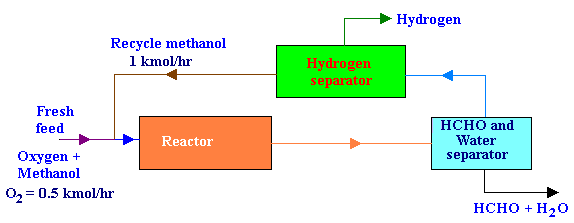### Recycle Reactor with Separators

Methanol vapor can be converted into formaldehyde by the following reaction scheme:

CH3OH + 0.5 O2 -> HCHO + H2O

CH3OH -> HCHO + H2

The fresh feed to the process was 0.5 kmol / hr of O2 and an excess methanol. All of the O2 reacts in the reactor. Formaldehyde and water are removed from the product stream first, after which H2 is removed from the recycled methanol. The recycle flow rate of methanol was 1 kmol/hr. The ratio of methanol reacting by decomposition to that by oxidation was 3. Draw the flow diagram and then calculate the per pass conversion of methanol in the reactor and the fresh feed rate of methanol.

Calculations:

The flow diagram for the above process is as given below:From the problem statements,

1. all of the O2 entering, reacts in the reactor and
2. the ratio of methanol reacting by decomposition to that by oxidation = 3.

The number of moles taking parts in the reactions are given below:

CH3OH + 0.5 O2 → HCHO + H2O   → 1

1              0.5              1         1

CH3OH → HCHO + H2   → 2

1              1          1

from reaction 1,

0.5 mole of O2 ≡ 1 mole of CH3OH

Therefore, for complete conversion of oxygen, 0.5 mole/hr of O2 needs 1 mole/hr of CH3OH.

And from the ratio of methanol conversion, methanol reacting by decomposition = 3 times that by oxidation = 3 mole/hr of CH3OH.

Therefore,

Total methanol entering the reactor = Methanol reacted by oxidation + methanol reacted by decomposition + recycle methanol = 1 + 3 + 1 = 5 kmol/hr.

Conversion per pass = 100 x (Total methanol reacted /Total methanol entering the reactor)

= 100 x 4/5 = 80%

Fresh methanol rate = Total methanol entering the reactor - recycle methanol = 5 - 1 = 4 kmol/hr.